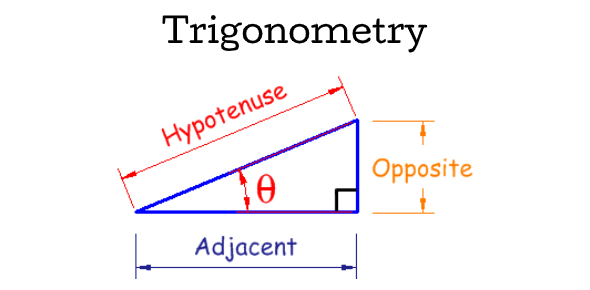# Trigonometry Quiz On Triangles! Math Trivia

10 Questions | Total Attempts: 281Settings.

Related Topics
• 1.
A right triangle doesn't contain a 90 degree angle.
• A.

True

• B.

False

• 2.
A scalene triangle has equal sides and angles.
• A.

True

• B.

False

• 3.
This is a  isometric triangle .
• A.

True

• B.

False

• 4.
There are two right angles in a right triangle.
• A.

True

• B.

False

• 5.
An isosceles has three equal sides
• A.

True

• B.

False

• 6.
The total interior angle measure for a triangle is 180 degrees.
• A.

True

• B.

False

• 7.
If a three sided figure has a 80,90, 90 angle measurement it is a triangle.
• A.

True

• B.

False

• 8.
In a equilateral triangle all angles are equal to?
• A.

456

• B.

230

• C.

420

• D.

60

• 9.
The equation to the pythagorean theorem is a+b=c
• A.

True

• B.

False

• 10.
In a scalene triangle, all angles are diffrerent
• A.

True

• B.

False